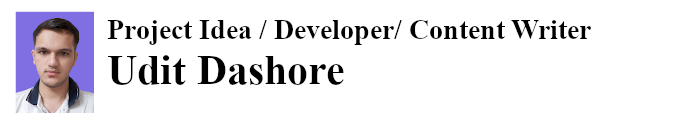| Technical Review: ABCOM Team | Level: Intermediate |

These days you would find many goodies on the Internet, one such goody is to detect the expression or the emotion of a person in a photograph. The following screenshot shows the emotions of a famous Indian actor analyzed by one such site - Emotion Recognition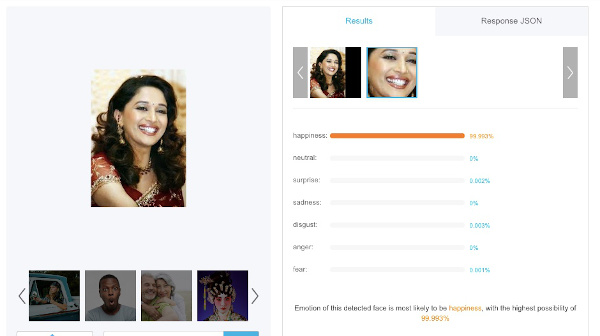Courtesy https://www.faceplusplus.com

This kind of application has many practical uses. One such use would be by the cab aggregators like Ola, Uber where they can monitor their drives in real time. The following picture shows one such example,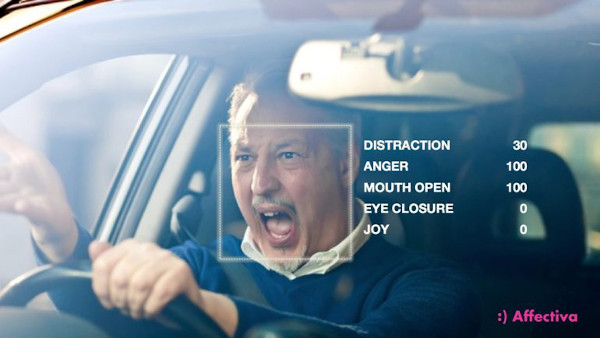Affectiva Automotive AI hopes to improve driver safety. (Credit: Affectiva)

Want to know how such sites analyze emotions. The current day Machine Learning models perform such tasks. With the deep neural networks, you can create such models and that is what you would learn in this short tutorial.

I assume you are familiar with the process of ML development and understand convolutional neural networks.

## Project Description

To train neural networks, what is essentially required is a proper dataset. For detecting emotions, obviously you would need lots of photos with each photo appropriately labeled to reflect the emotion. Fortunately, Kaggle has made such a dataset available where the emotions are classified under 7 categories, such as happy, angry, fear, and so on. Each category has several images. You will use this dataset to train the network.

## Creating Project

You will use Google Colab to create this project. Do not know how to use Colab? Here is a short tutorial. Create a new notebook and rename it EmotionRecognizer.

Import the required libraries.

``````import numpy as np
import matplotlib.pyplot as plt
import os
%matplotlib inline

from tensorflow.keras.preprocessing.image import ImageDataGenerator
from tensorflow.keras.layers import Dense, Input, Dropout,Flatten, Conv2D
from tensorflow.keras.layers import BatchNormalization, Activation, MaxPooling2D
from tensorflow.keras.models import Model, Sequential
from IPython.display import Image
import tensorflow as tf
from skimage.color import rgb2lab,gray2rgb,rgb2gray
from skimage.transform import resize

from tqdm import tqdm
import IPython.display as display
from PIL import Image
``````

``````    !wget http://abcom.com/article/Project.rar
!unrar x 'Project.rar'
``````

You will now have two folders test and train, each containing seven subfolders. Each subfolder has several sample images. The folder structure is shown here:### Exploring the Dataset

We will now print a sample image from each category and also get a count of the total number of images in each.

``````    img = []
for expression in os.listdir("/content/train/"):
i=0
print('\n'+str(len(os.listdir("/content/train/" + expression))) + " " + expression + " images")
train_ids = next(os.walk("/content/train/" + expression+'/'))
for n, id_ in tqdm(enumerate(train_ids), total=len(train_ids)):
path = "/content/train/" + expression+'/' + id_+''
#if i<1:
img.append((img1,expression))
i=i+1
``````

After running this code you will know that the dataset contains 436 disgust, 3171 surprise, 7214 happy, 4695 neutral, 4097 fear, 3995 angry and 4380 sad images.

The following code prints a sample image in each category:

``````    fig = plt.figure(figsize=(12,12))
ax1.set_title(img)
ax1.imshow(img)
ax2.imshow(img)
ax2.set_title(img)
ax3.imshow(img)
ax3.set_title(img)
ax4.imshow(img)
ax4.set_title(img)
ax5.imshow(img)
ax5.set_title(img)
ax6.imshow(img)
ax6.set_title(img)
ax7.imshow(img)
ax7.set_title(img)
``````

The output below shows an image in each category.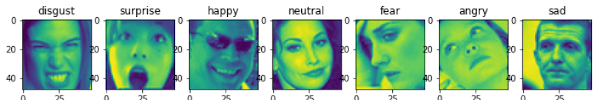### Generating the Training Batches

We now create a training dataset. You will use the preprocessing library of the tf.keras to modify each image in the training set using the ImageDataGenerator function for better training. The images are rotated, shifted, flipped and zoomed in certain predefined ranges. Each image is then resized to 48x48 and converted to grayscale. The batches of data are created after shuffling the images in the set.

``````    img_size = 48
batch_size = 64
datagen_train = ImageDataGenerator(
shear_range=0.2,
zoom_range=0.2,
rotation_range=20,
horizontal_flip=True)

train_generator = datagen_train.flow_from_directory("/content/train/",
target_size=(img_size,img_size),
color_mode="grayscale",
batch_size=batch_size,
class_mode='categorical',
shuffle=True)
``````

### Defining Model

We define the model as follows:

``````    def  createModel():
model = Sequential()

return model
``````

The input to the model is of the shape (48,48,1) which indicates that we are inputting the grayscale image to our model.

### Creating Model

You create model and print its summary using the following statements:

``````    model = createModel()
model.summary()
``````

Here is the model summary: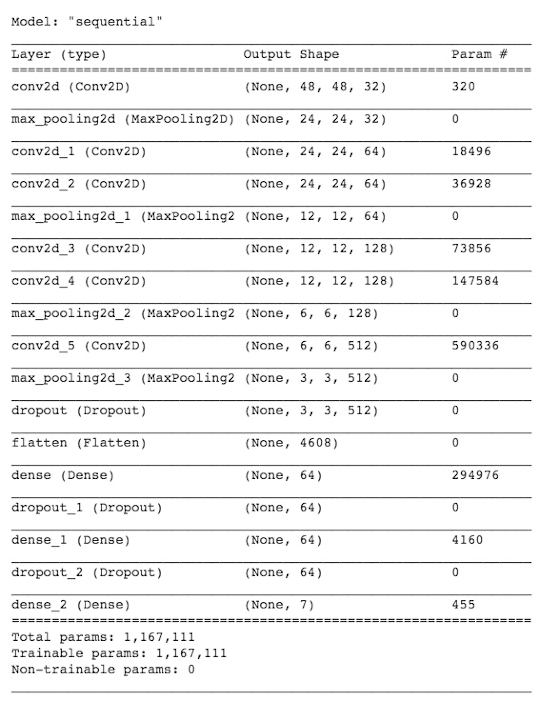The model has almost 1.1 million trainable parameters.

Print the model plot for visualization.

``````    tf.keras.utils.plot_model(model)
``````

The plot is shown here: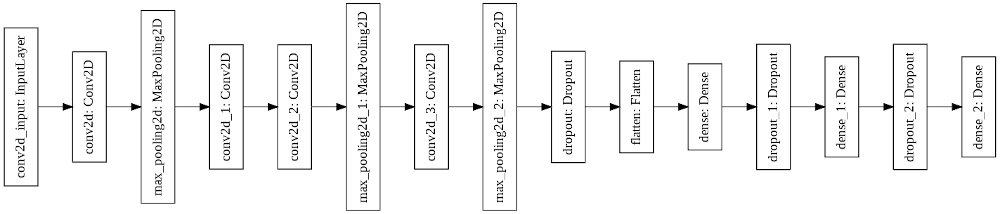### Model Compiling

You compile the model by calling its compile method:

``````    model.compile(optimizer='adam', loss='categorical_crossentropy', metrics=['accuracy'])
``````

We used adam optimizer and categorical crossentropy for the loss function.

### Model Training

You train the model by calling its fit method.

``````    history = model.fit(
x=train_generator,
epochs=25,
)
``````

The model is trained for 25 epochs, which is usually not sufficient training for producing good results. It took me about 27 seconds for each epoch on a GPU.

### Testing the Model on the Test Data

Prepare the test data in the same way as you did for the train data, except for the image augmentation.

``````    test_img = []
test_exp = []
for expression in os.listdir("/content/test/"):
train_ids = next(os.walk("/content/test/" + expression+'/'))
for n, id_ in tqdm(enumerate(train_ids), total=len(train_ids)):
path = "/content/test/" + expression+'/' + id_+''
img = gray2rgb(rgb2gray(img))
img = rgb2lab(img)[:,:,0]
test_img.append(img)
test_exp.append(expression)

test_img = np.asarray(test_img)
test_exp = np.asarray(test_exp)
test_img = test_img.reshape(test_img.shape+(1,))
``````

Here , we save each image in the test_img list and the corresponding expression in the test_exp list.

### Inference

We infer the model using the above prepared test dataset.

``````    y_pred = model.predict(test_img)
``````

The result stored in the y_pred is in the form of the probabilities of the different classes. We need to select the class with the maximum probability and map our expression to it. This is done in the following code:

``````    pred_class = []
for i in  range (len(y_pred)):
pr = y_pred[i].argmax()
pred_class.append(pr)

emotion = {0:'angry', 1:'disgust', 2:'fear', 3:'happy',
pred_emo = []
for i in  range(len(pred_class)):
pred_emo.append(emotion[pred_class[i]])
``````

We will print the prediction results in a bar plot that shows the real and the predicted values side by side for each category.

``````    label = ['angry','disgust','fear','happy','neutral', 'sad','surprise']
test_class = [0,0,0,0,0,0,0]
for i in  range (len(test_exp)):
if test_exp[i]=='angry':
test_class = test_class+1
if test_exp[i]=='disgust':
test_class = test_class+1
if test_exp[i]=='fear':
test_class = test_class+1
if test_exp[i]=='happy':
test_class = test_class+1
if test_exp[i]=='neutral':
test_class = test_class+1
test_class = test_class+1
if test_exp[i]=='surprise':
test_class = test_class+1
test_class

pred_class = [0,0,0,0,0,0,0]
for i in  range (len(pred_emo)):
if pred_emo[i]=='angry':
pred_class = pred_class+1
if pred_emo[i]=='disgust':
pred_class = pred_class+1
if pred_emo[i]=='fear':
pred_class = pred_class+1
if pred_emo[i]=='happy':
pred_class = pred_class+1
if pred_emo[i]=='neutral':
pred_class = pred_class+1
pred_class = pred_class+1
if pred_emo[i]=='surprise':
pred_class = pred_class+1
pred_class

x = np.arange(len(label)) # the label locations
width = 0.35  # the width of the bars

fig, ax = plt.subplots()
rects1 = ax.bar(x - width/2, pred_class, width, label='Predicted')
rects2 = ax.bar(x + width/2, test_class, width, label='Original')
ax.set_ylabel('Value')
ax.set_xticks(x)
ax.set_xticklabels(label)
ax.legend()
``````

The output is shown here: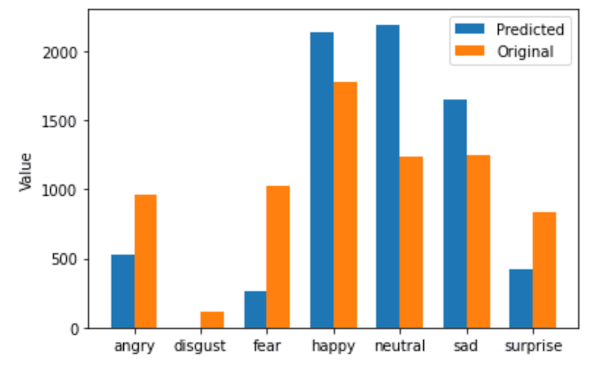You may try for more number of training epochs or play around with the model’s hyperparameters to improve performance.

## Testing on Unseen Images

As a last exercise in this we are going to predict the model on some unseen images picked up from the web. Download the images using following code:

``````    !wget = 'https://raw.githubusercontent.com/abcom-mltutorials/emotionsdetector/master/emotion1.jpg'
!wget = 'https://raw.githubusercontent.com/abcom-mltutorials/emotionsdetector/master/emotion2.jpg'
!wget = 'https://raw.githubusercontent.com/abcom-mltutorials/emotionsdetector/master/emotion3.jpg'
!wget = 'https://raw.githubusercontent.com/abcom-mltutorials/emotionsdetector/master/emotion4.jpg'
!wget = 'https://raw.githubusercontent.com/abcom-mltutorials/emotionsdetector/master/emotion5.jpg'
``````

The images must undergo the same preprocessing as we did for test images, before we ask the model to infer on those.

``````    l=['emotion1.jpg','emotion2.jpg','emotion3.jpg','emotion4.jpg','emotion5.jpg']
exp = []
for i in l:
img = resize(img, (48, 48), mode='constant', preserve_range=True)
img = gray2rgb(rgb2gray(img))
img = rgb2lab(img)[:,:,0]
img = img.reshape((1,)+img.shape+(1,))
result = model.predict(img)
exp.append(emotion[result.argmax()])
``````

Finally, we display the prediction result using following code:

``````    fig = plt.figure(figsize=(12,12))
ax1.set_title(exp)
ax1.imshow(im,cmap="gray")
ax1.axis('off')
ax2.imshow(im)
ax2.set_title(exp)
ax2.axis('off')
ax3.imshow(im)
ax3.set_title(exp)
ax3.axis('off')
ax4.imshow(im)
ax4.set_title(exp)
ax4.axis('off')
ax5.imshow(im)
ax5.set_title(exp)
ax5.axis('off')
``````

#### Output: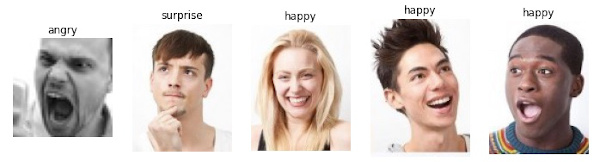### Summary

In this text you studied the usage of keras model for prediction the Facial expression from the image.You implemented a deep neural network model for Facial expression Recognition. To improve the quality of prediction you also used some inbuilt preprocessing methods.
Hope you enjoyed the course.